# Derivative Application: Deriving the Velocity from the Distance Function

in MES Science4 months ago (edited)

In this video I go through an example of using the derivative rules I showed in my earlier videos. I look at determining the velocity and distance traveled of a random particle with a distance function. The slope or instantaneous change in distance is the velocity and I use the derivative to derive the velocity equation from the distance function. This is a very useful example and shows how useful calculus is in real world problems.

### View Video Notes Below!

View these notes as an article: https://peakd.com/@mes
Subscribe via email: http://mes.fm/subscribe
Donate! :) https://mes.fm/donate
Subscribe to MES Truth: https://mes.fm/truth

Reuse of my videos:

• Feel free to make use of / re-upload / monetize my videos as long as you provide a link to the original video.

Fight back against censorship:

• Bookmark sites/channels/accounts and check periodically
• Remember to always archive website pages in case they get deleted/changed.

Recommended Books:

Join my forums!

Follow along my epic video series:

NOTE #1: If you don't have time to watch this whole video:

Browser extension recommendations:

# Derivative Applications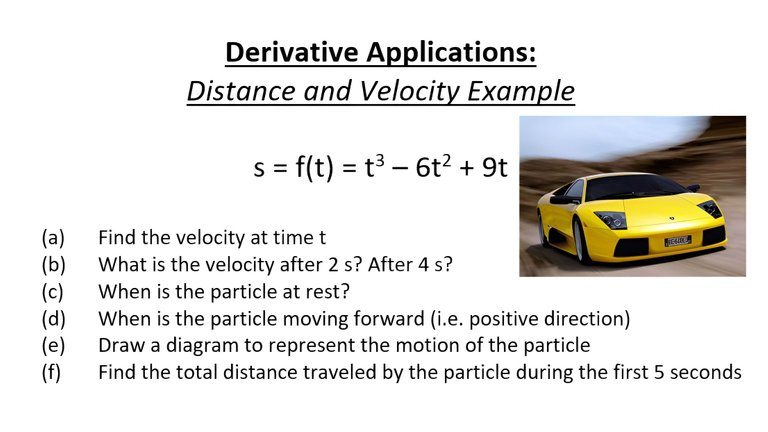# Distance and Velocity Example

Solve the following questions, given the distance formula of a particle below:

s = f(t) = t3 – 6t2 + 9t

(a) Find the velocity at time t
(b) What is the velocity after 2 s? After 4 s?
(c) When is the particle at rest?
(d) When is the particle moving forward (i.e. positive direction)
(e) Draw a diagram to represent the motion of the particle
(f) Find the total distance traveled by the particle during the first 5 seconds

## Solution to (a)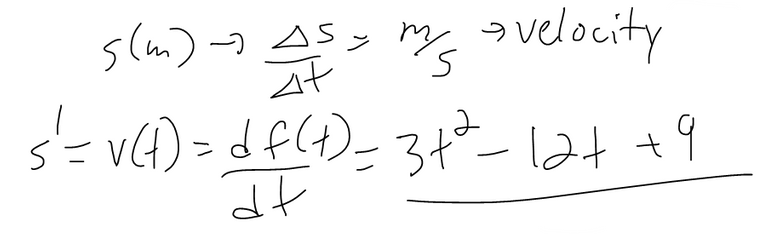## Solution to (b)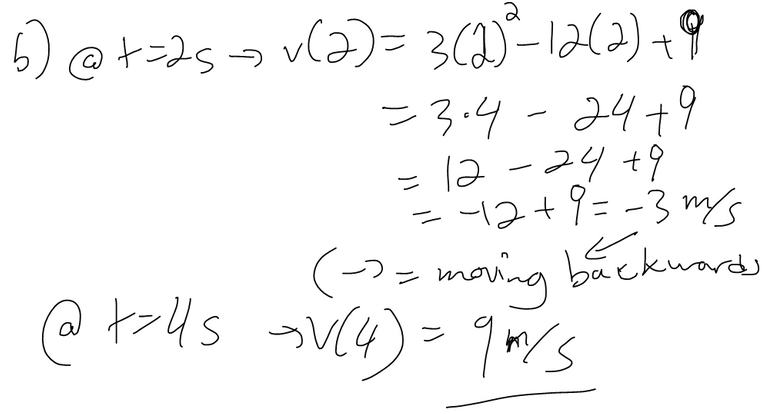## Solution to (c)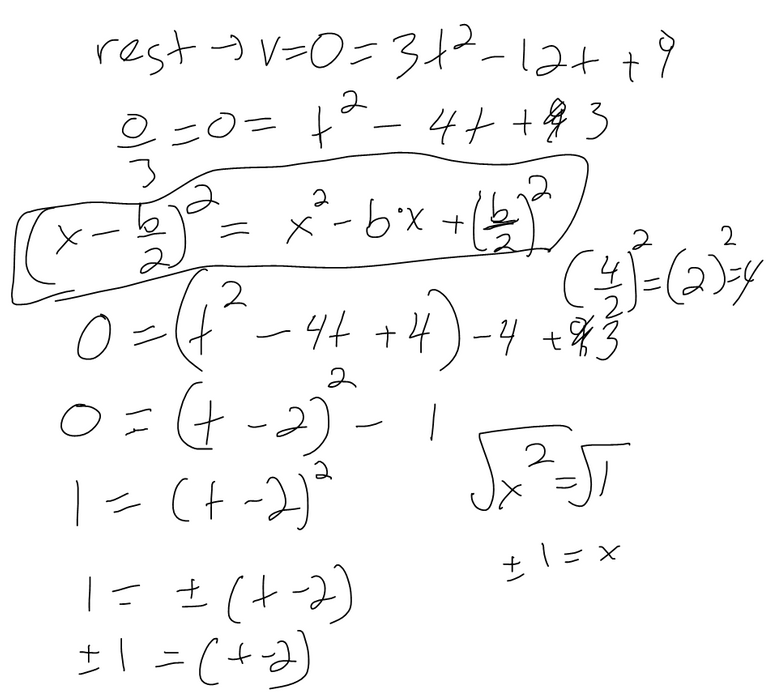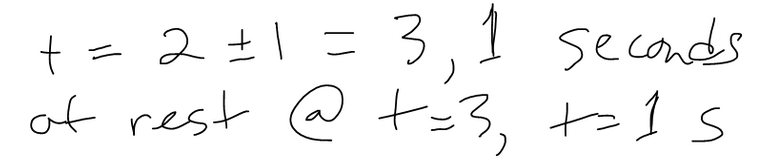## Solution to (d)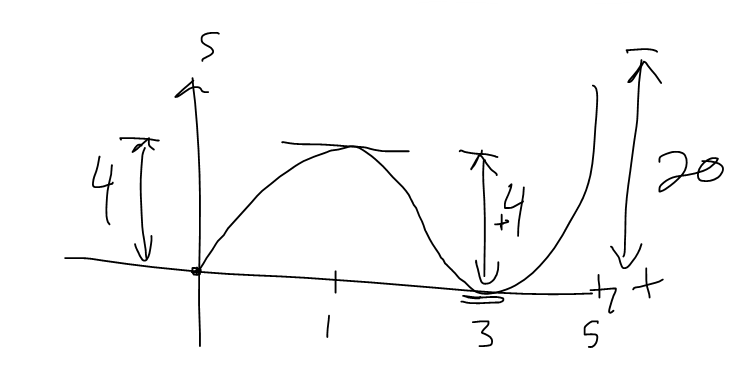## Solution to (e)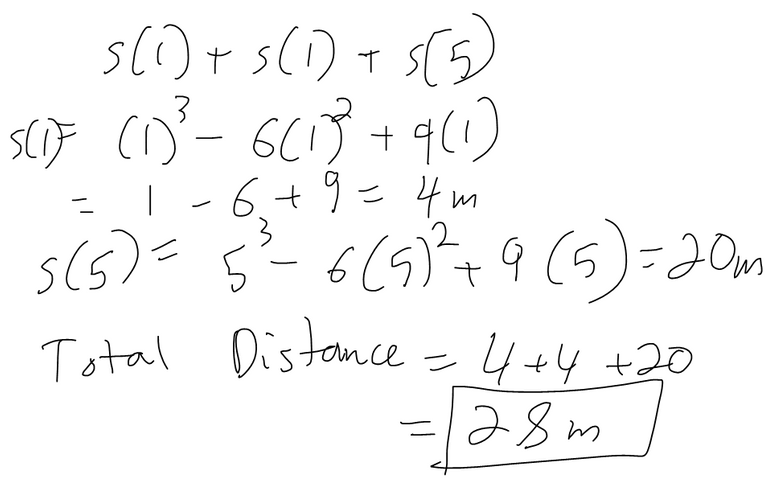Sort: